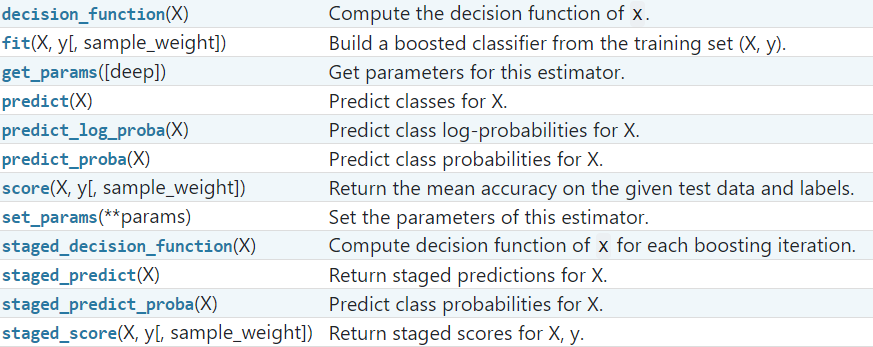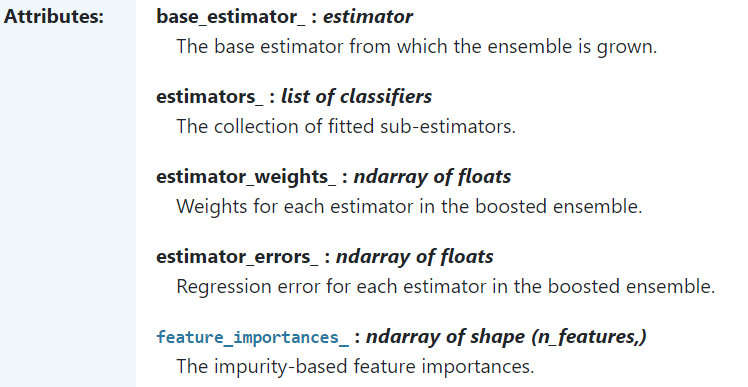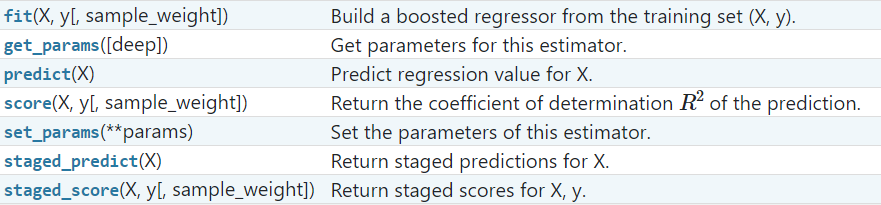#1147-柳同学

## 热门标签

, , , ,

sklearn.ensemble.AdaBoostClassifier(base_estimator=None, *, n_estimators=50, learning_rate=1.0,
algorithm='SAMME.R', random_state=None)


base_estimator_ :estimator

estimators_ : list of classifiers

classes_ : ndarray of shape (n_classes,)

n_classes_ : int

estimator_weights_ : ndarray of floats

estimator_errors_ : ndarray of floatssklearn.ensemble.AdaBoostRegressor(base_estimator=None, *, n_estimators=50, learning_rate=1.0,
loss='linear', random_state=None)### 参数介绍

base_estimator : object, default=None

n_estimators : int, default=50

learning_rate : float, default=1.

algorithm : {‘SAMME’, ‘SAMME.R’}, default=’SAMME.R’

loss : {‘linear’, ‘square’, ‘exponential’}, default=’linear’

random_state : int, RandomState instance or None, default=None

### 评论 抢沙发### 觉得文章有用就打赏一下文章作者

#### 支付宝扫一扫打赏#### 微信扫一扫打赏Vieu3.3主题Q Q 登 录